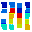# Shenzhen Bingchuan Network Co Ltd SZSE:300533Shenzhen Bingchuan Network Co Ltd
SZSE:300533
Price: 29.57 CNY -1.04%
Updated:

## DCF Value

This DCF valuation model was created byAlpha Spread and was last updated on Nov 25, 2023.

Estimated DCF Value of one300533 stock is 65.69 CNY. Compared to the current market price of 29.57 CNY, the stock is Undervalued by 55%.

DCF Valuation FAQ:
DCF Value
Base Case
65.69 CNY
Undervaluation 55%
DCF Value
Price65.69
DCF Value
Shenzhen Bingchuan Network Co Ltd Competitors:
DCF Valuation
002602
Zhejiang Century Huatong Group Co Ltd
800
A8 New Media Group Ltd
603258
Hangzhou Electronic Soul Network Technology Co Ltd
002624
Perfect World Co Ltd
300518
Shen Zhen Shengxunda Technology Co Ltd
600633
Zhejiang Daily Digital Culture Group Co Ltd

Revenue
3.5B 15.2B
Operating Income
159m 1.1B
FCFF
135m 992m

## Present Value Calculation

The first step in the DCF calculation process is to estimate the company's future free cash flow. Once free cash flow is forecasted, it is discounted at a risk-appropriate discount rate. The resulting value is the present value of the company's free cash flow. You can change any model inputs below.

Available Valuation Models
1 valuation model availableUpdated on Nov 25, 2023
Default Valuation
Other investors haven't shared any valuation models for Shenzhen Bingchuan Network Co Ltd.
Model Settings
Operating Model
Discount Rate
6.59%
Forecast Period
5 Years
Terminal Growth
0%
Discount Rate
6.59%
Terminal Growth
0%
Growth Period
5 Years

### DCF Model Base Case ScenarioDCF Model
Currency: CNY
Millions

To view the process of deriving the DCF Value of one share from the estimated Present Value, see the DCF Value Calculation block.

## DCF Value Calculation

Depending on which type of operating model is chosen (equity or whole firm valuation model), the resulting value is either the value of equity or the value of the entire firm. In the case of the latter, to move from the value of the firm to the value of equity, liabilities are subtracted and assets are added.

### Capital Structure From Present Value to DCF ValuePresent Value 12.7B CNY + Cash & Equivalents 737m CNY + Investments 1.7B CNY Firm Value 15.2B CNY - Debt 17.5m CNY - Minority Interest -42.4m CNY Equity Value 15.2B CNY / Shares Outstanding 231m 300533 DCF Value 65.69 CNY
Undervalued by 55%

To view the process of calculating the Present Value of Shenzhen Bingchuan Network Co Ltd' future free cash flow, see the Present Value Calculation block.

Estimated DCF Value of one300533 stock is 65.69 CNY. Compared to the current market price of 29.57 CNY, the stock is Undervalued by 55%.
1. Present Value Calculation. Using the DCF Operating Model we projectShenzhen Bingchuan Network Co Ltd's future free cash flow and discount it at a selected discount rate to calculate its Present Value (12.7B CNY).
2. DCF Value Calculation. We use the company's capital structure to calculate the total Equity Value based on the previously computed Present Value of the free cash flow. Dividing the Equity Value by the number of shares outstanding gives us the DCF Value of 65.69 CNY per one300533 share.